Function Repository Resource:

# MoleculeSymmetryPlot3D

Show a molecule in 3D together with its symmetry elements

Contributed by: Jason Biggs
 ResourceFunction["MoleculeSymmetryPlot3D"][mol] returns a Manipulate combining a 3D plot of the Molecule mol together with graphical depictions of its symmetry elements.

## Details and Options

Symmetry elements are displayed with the following Graphics3D primitives:
 Tube rotation axes Sphere inversion centers Hyperplane reflection panes

## Examples

### Basic Examples (2)

Tetramethyl ammonium has Td symmetry:

 In:=Out=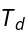Visualize the symmetry elements present:

 In:=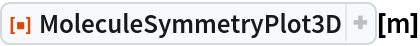Out=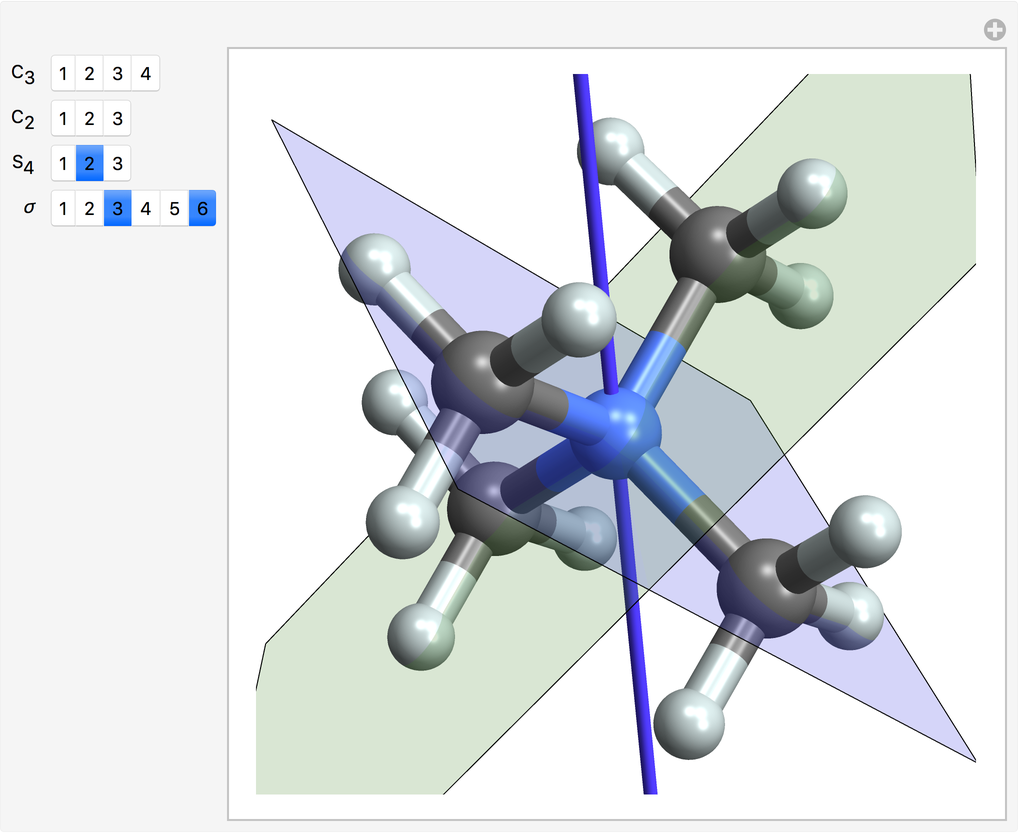Visualize the C2 rotation axes in benzene:

 In:=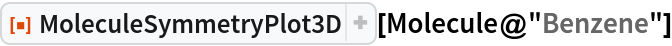Out=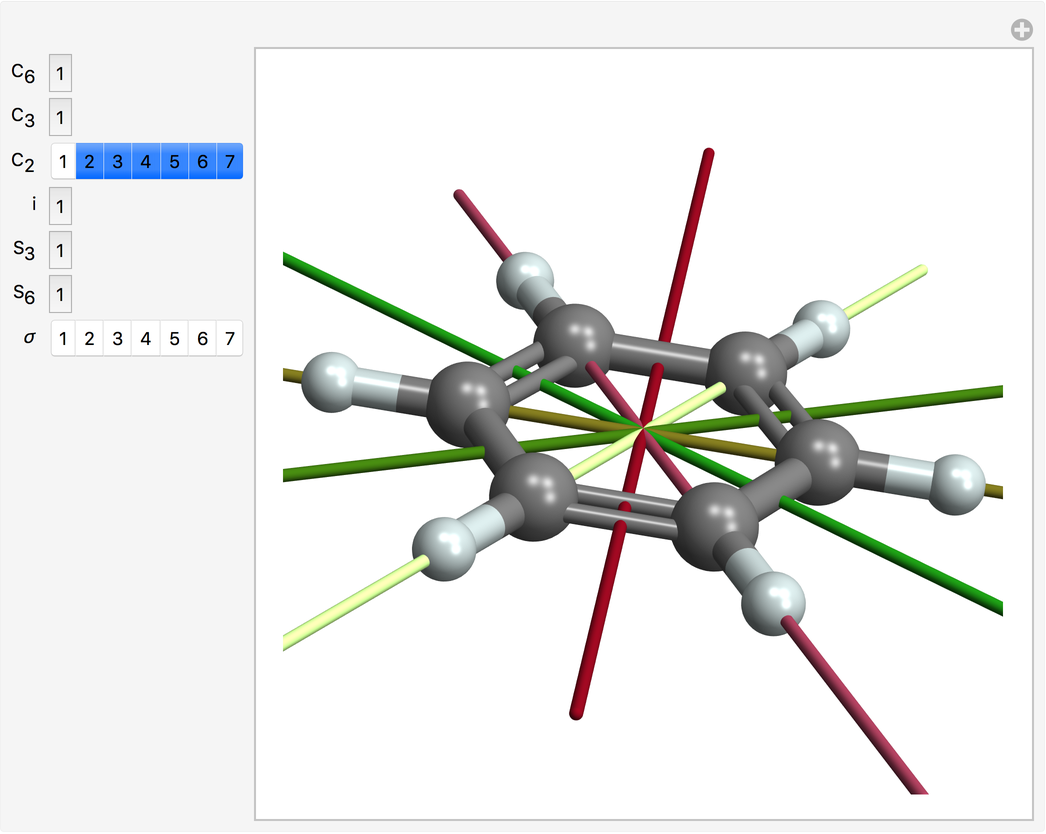### Possible Issues (2)

If a molecule's coordinates are not symmetric, no symmetry elements will be found:

 In:=Out=In:=Out=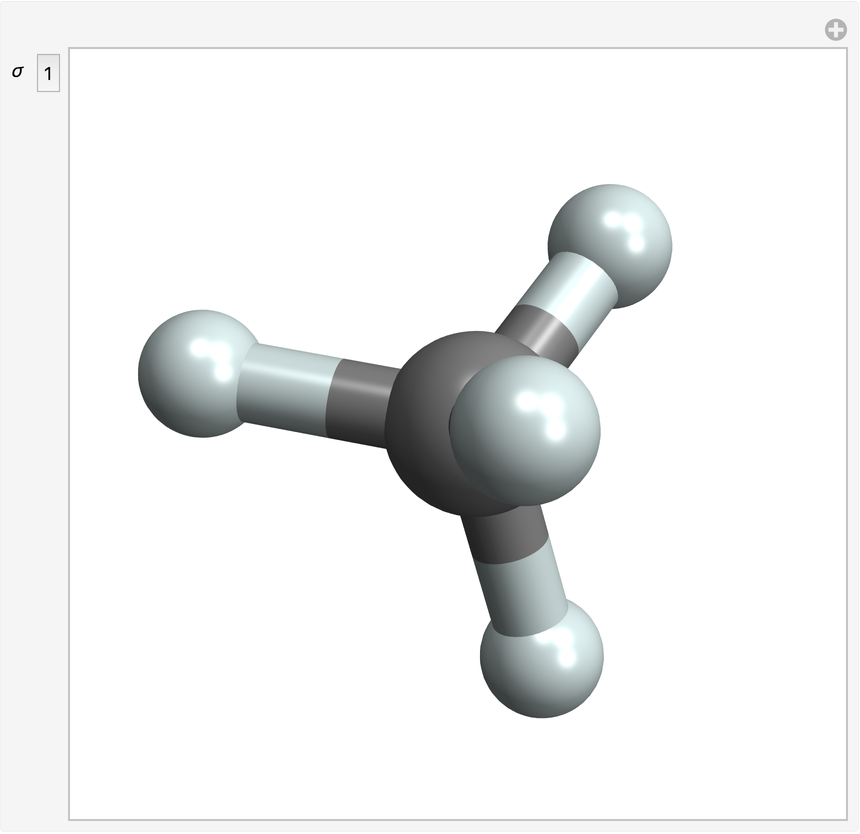Use the Merck molecular force field to find coordinates with higher symmetry:

 In:=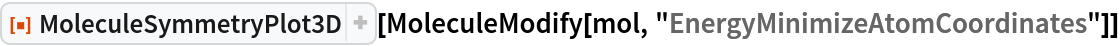Out=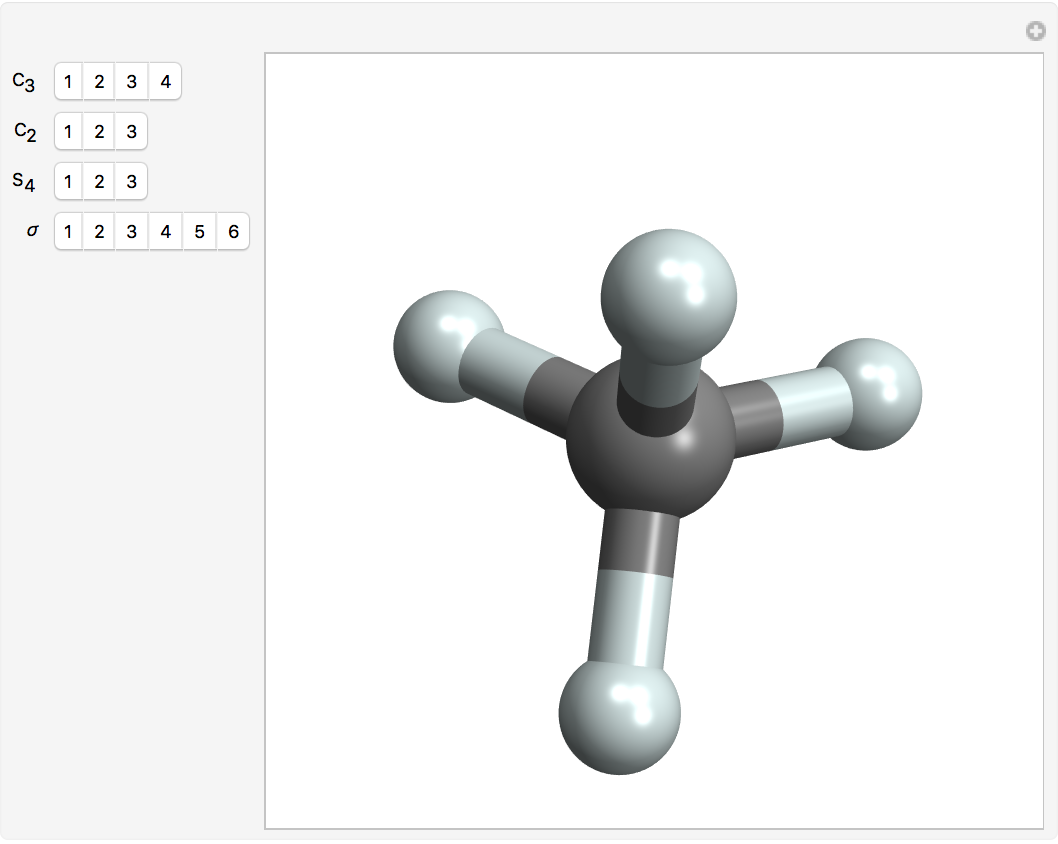JasonB

## Version History

• 1.0.0 – 05 August 2020